Скачать презентацию User-defined Structure Types Structures collection of data

39b93e4e1d81b7dc851802720c7f4e60.ppt

• Количество слайдов: 39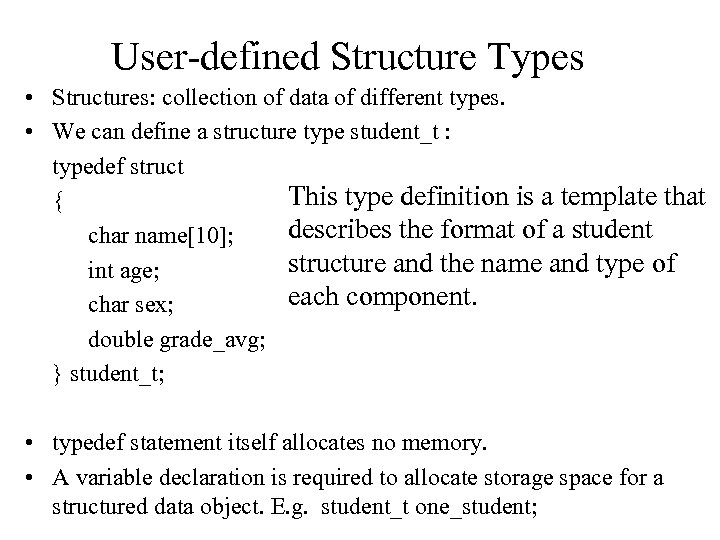User-defined Structure Types • Structures: collection of data of different types. • We can define a structure type student_t : typedef struct This type definition is a template that { describes the format of a student char name; structure and the name and type of int age; each component. char sex; double grade_avg; } student_t; • typedef statement itself allocates no memory. • A variable declaration is required to allocate storage space for a structured data object. E. g. student_t one_student;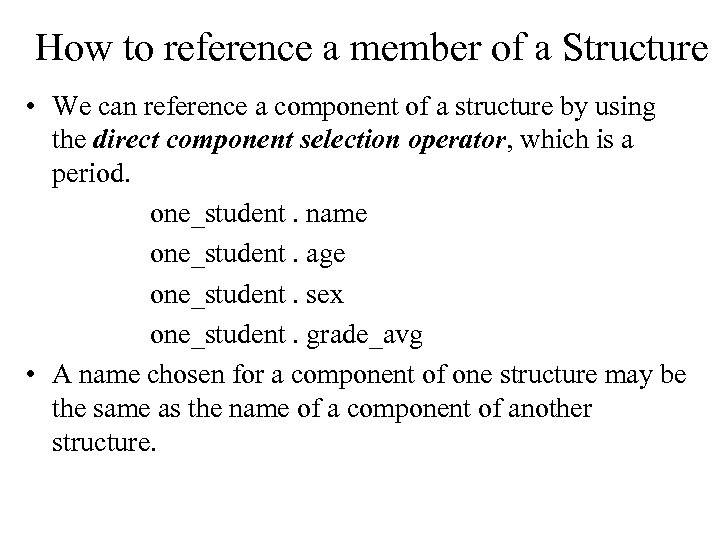How to reference a member of a Structure • We can reference a component of a structure by using the direct component selection operator, which is a period. one_student. name one_student. age one_student. sex one_student. grade_avg • A name chosen for a component of one structure may be the same as the name of a component of another structure.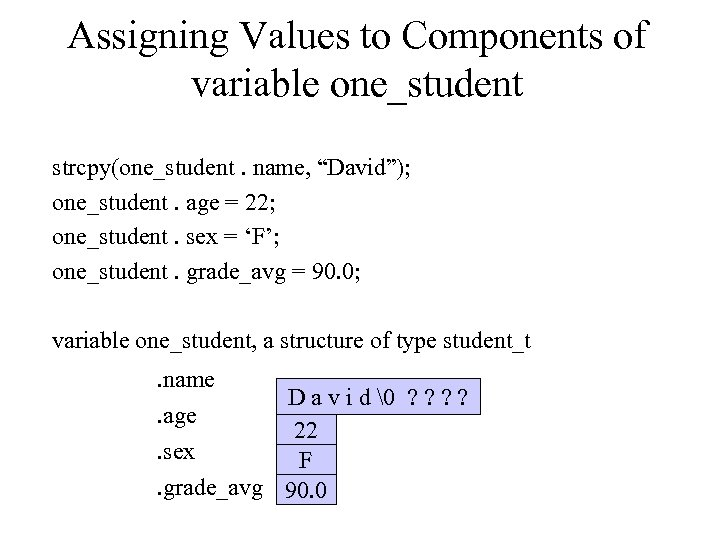Assigning Values to Components of variable one_student strcpy(one_student. name, “David”); one_student. age = 22; one_student. sex = ‘F’; one_student. grade_avg = 90. 0; variable one_student, a structure of type student_t. name. age. sex D a v i d ? ? 22 F. grade_avg 90. 0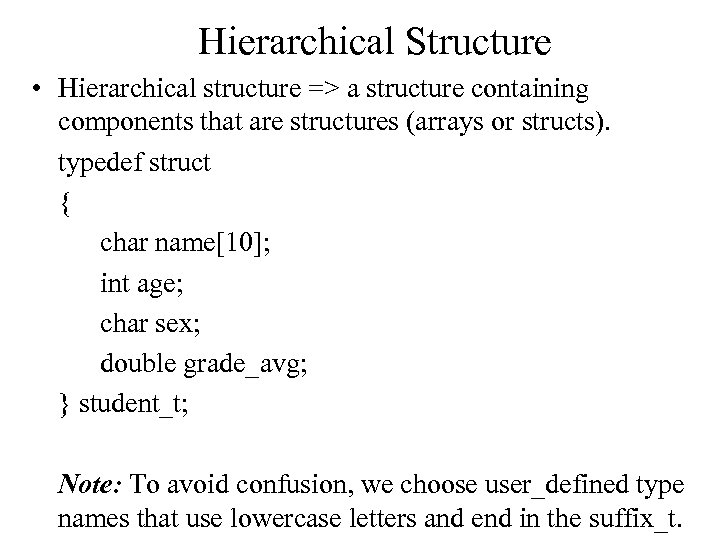Hierarchical Structure • Hierarchical structure => a structure containing components that are structures (arrays or structs). typedef struct { char name; int age; char sex; double grade_avg; } student_t; Note: To avoid confusion, we choose user_defined type names that use lowercase letters and end in the suffix_t.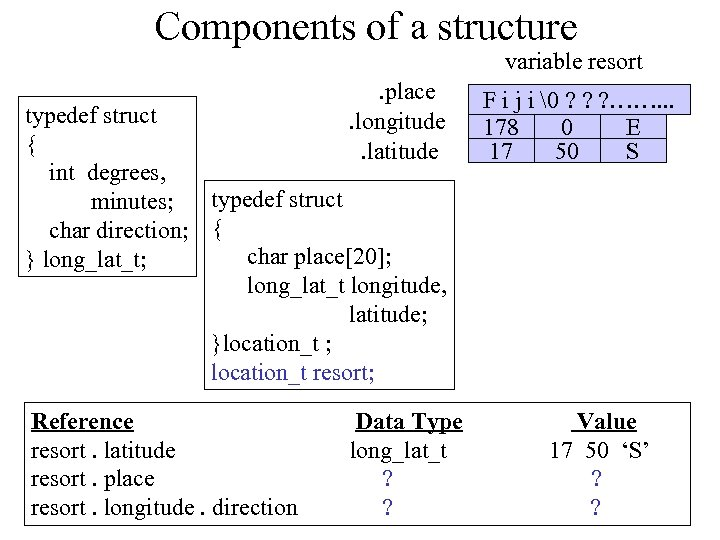Components of a structure variable resort. place. longitude. latitude typedef struct { int degrees, typedef struct minutes; char direction; { char place; } long_lat_t; long_lat_t longitude, latitude; }location_t ; location_t resort; Reference resort. latitude resort. place resort. longitude. direction Data Type long_lat_t ? ? F i j i ? ? ? ……. . . 178 0 E 17 50 S Value 17 50 ‘S’ ? ?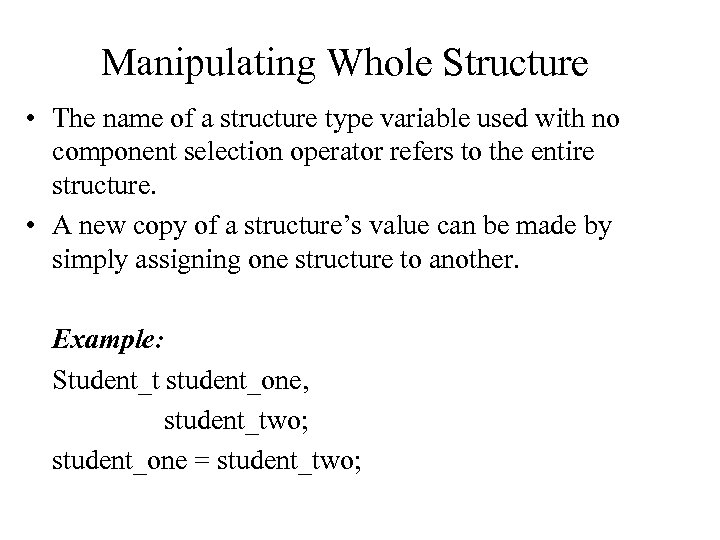Manipulating Whole Structure • The name of a structure type variable used with no component selection operator refers to the entire structure. • A new copy of a structure’s value can be made by simply assigning one structure to another. Example: Student_t student_one, student_two; student_one = student_two;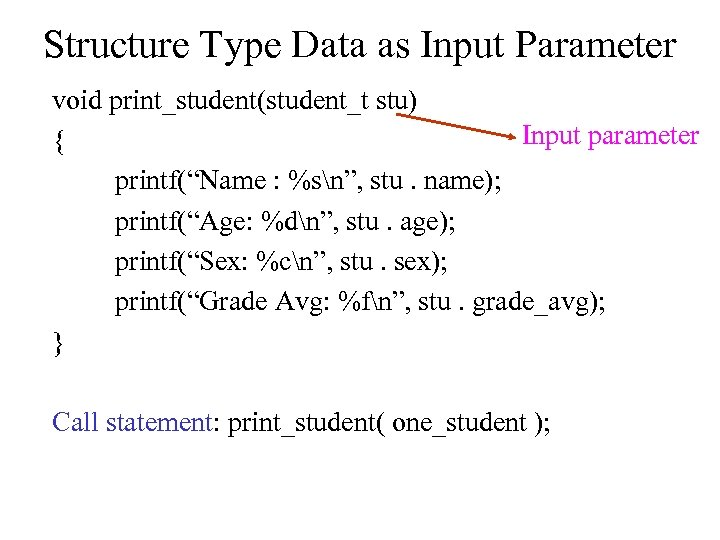Structure Type Data as Input Parameter void print_student(student_t stu) Input parameter { printf(“Name : %sn”, stu. name); printf(“Age: %dn”, stu. age); printf(“Sex: %cn”, stu. sex); printf(“Grade Avg: %fn”, stu. grade_avg); } Call statement: print_student( one_student );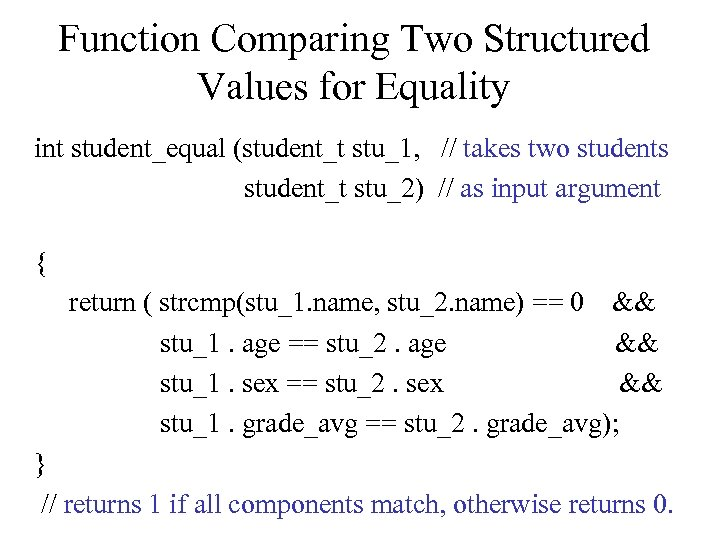Function Comparing Two Structured Values for Equality int student_equal (student_t stu_1, // takes two students student_t stu_2) // as input argument { return ( strcmp(stu_1. name, stu_2. name) == 0 && stu_1. age == stu_2. age && stu_1. sex == stu_2. sex && stu_1. grade_avg == stu_2. grade_avg); } // returns 1 if all components match, otherwise returns 0.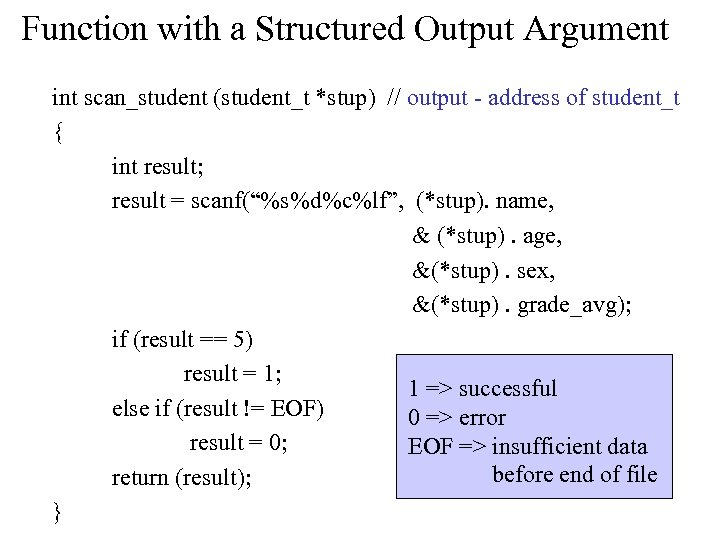Function with a Structured Output Argument int scan_student (student_t *stup) // output - address of student_t { int result; result = scanf(“%s%d%c%lf”, (*stup). name, & (*stup). age, &(*stup). sex, &(*stup). grade_avg); if (result == 5) result = 1; 1 => successful else if (result != EOF) 0 => error result = 0; EOF => insufficient data before end of file return (result); }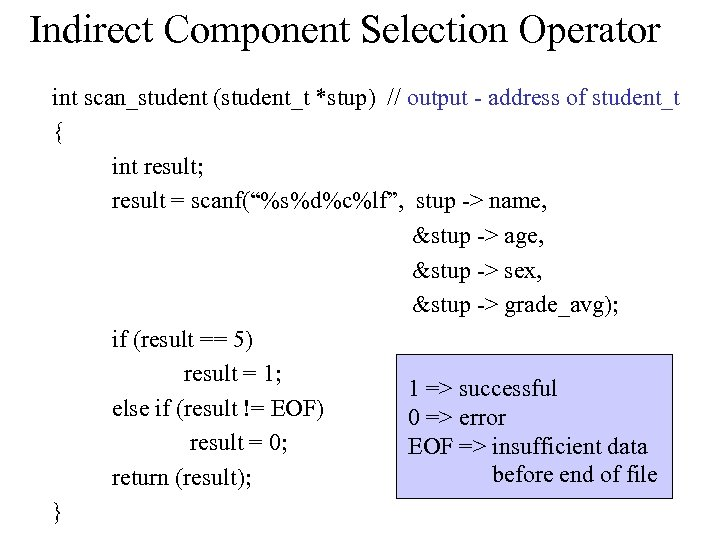Indirect Component Selection Operator int scan_student (student_t *stup) // output - address of student_t { int result; result = scanf(“%s%d%c%lf”, stup -> name, &stup -> age, &stup -> sex, &stup -> grade_avg); if (result == 5) result = 1; 1 => successful else if (result != EOF) 0 => error result = 0; EOF => insufficient data before end of file return (result); }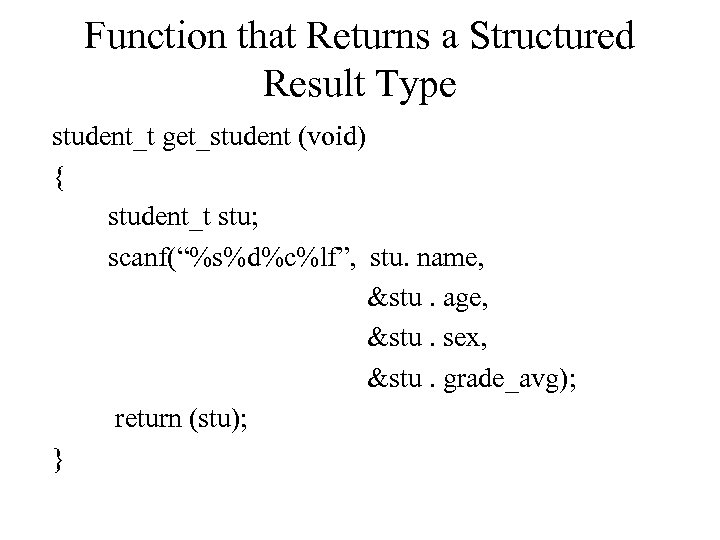Function that Returns a Structured Result Type student_t get_student (void) { student_t stu; scanf(“%s%d%c%lf”, stu. name, &stu. age, &stu. sex, &stu. grade_avg); return (stu); }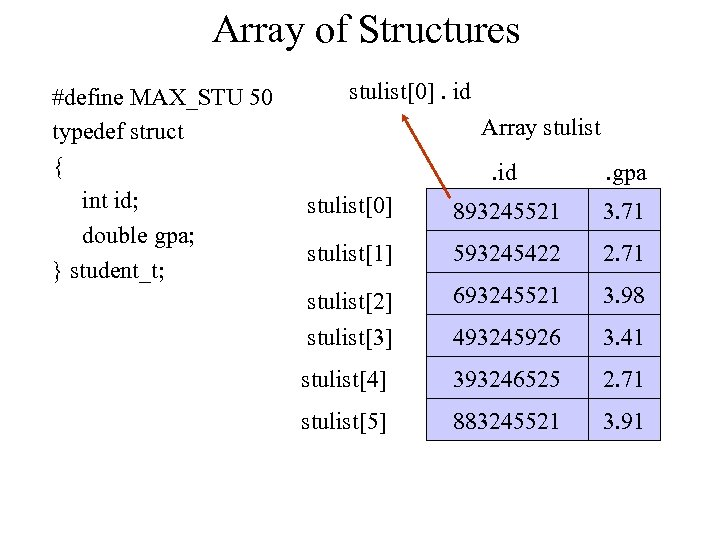Array of Structures #define MAX_STU 50 typedef struct { int id; double gpa; } student_t; stulist. id Array stulist. id . gpa stulist 893245521 3. 71 stulist 593245422 2. 71 stulist stulist 693245521 3. 98 493245926 3. 41 stulist 393246525 2. 71 stulist 883245521 3. 91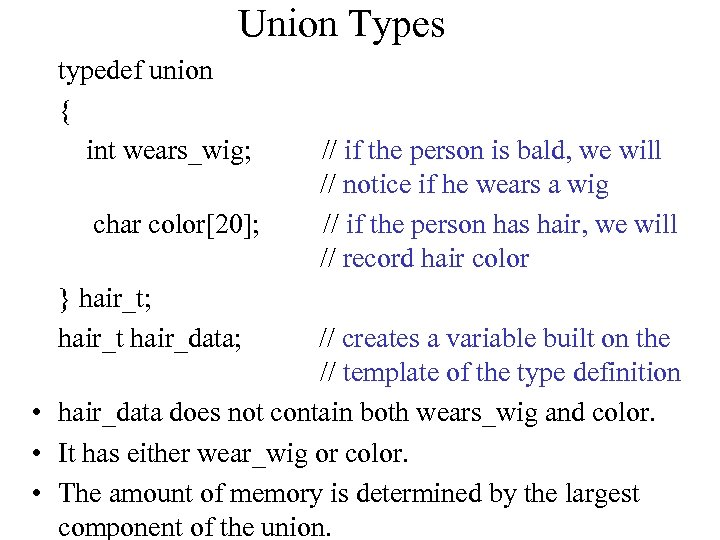Union Types typedef union { int wears_wig; char color; } hair_t; hair_t hair_data; // if the person is bald, we will // notice if he wears a wig // if the person has hair, we will // record hair color // creates a variable built on the // template of the type definition • hair_data does not contain both wears_wig and color. • It has either wear_wig or color. • The amount of memory is determined by the largest component of the union.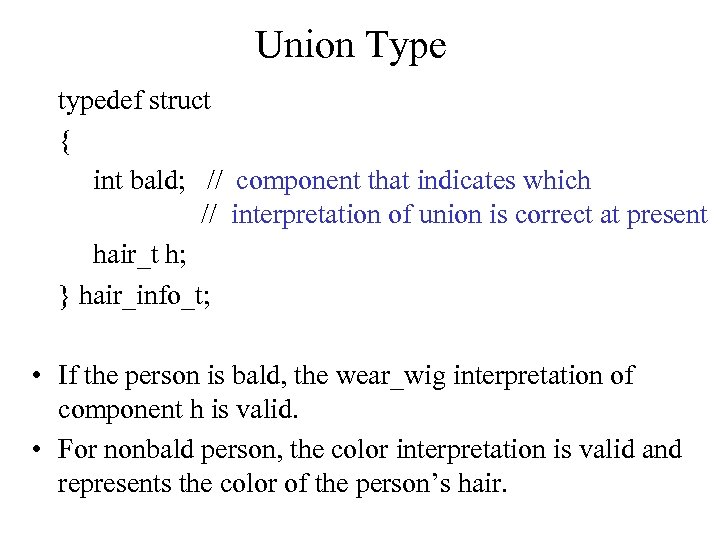Union Type typedef struct { int bald; // component that indicates which // interpretation of union is correct at present hair_t h; } hair_info_t; • If the person is bald, the wear_wig interpretation of component h is valid. • For nonbald person, the color interpretation is valid and represents the color of the person’s hair.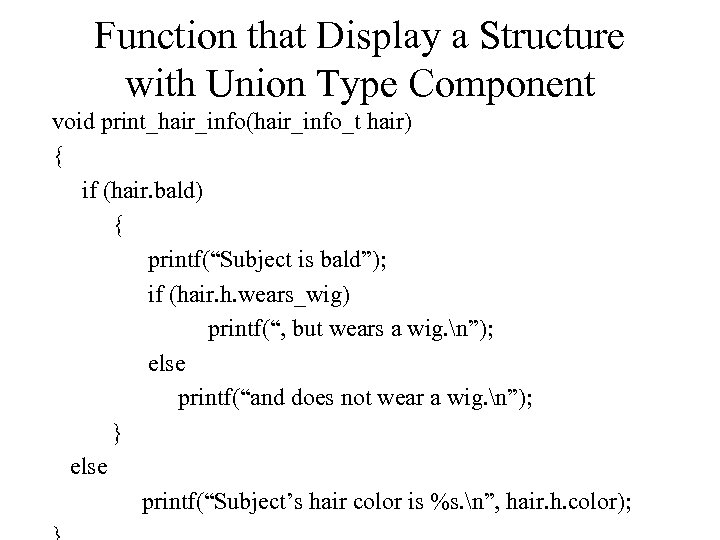Function that Display a Structure with Union Type Component void print_hair_info(hair_info_t hair) { if (hair. bald) { printf(“Subject is bald”); if (hair. h. wears_wig) printf(“, but wears a wig. n”); else printf(“and does not wear a wig. n”); } else printf(“Subject’s hair color is %s. n”, hair. h. color);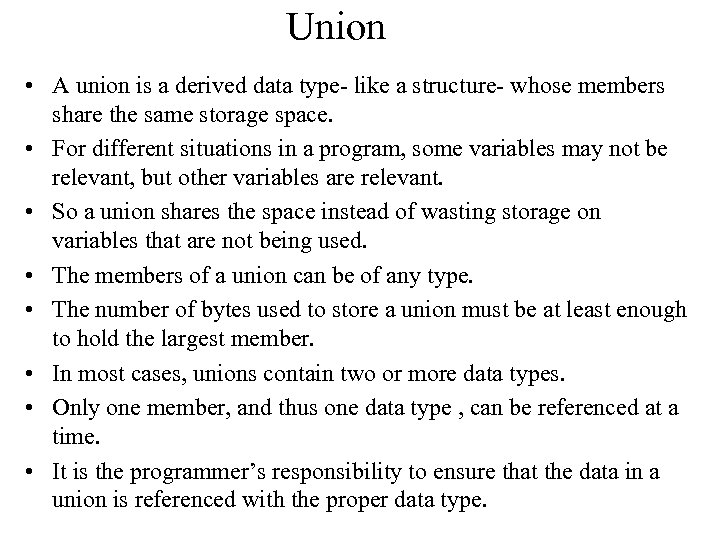Union • A union is a derived data type- like a structure- whose members share the same storage space. • For different situations in a program, some variables may not be relevant, but other variables are relevant. • So a union shares the space instead of wasting storage on variables that are not being used. • The members of a union can be of any type. • The number of bytes used to store a union must be at least enough to hold the largest member. • In most cases, unions contain two or more data types. • Only one member, and thus one data type , can be referenced at a time. • It is the programmer’s responsibility to ensure that the data in a union is referenced with the proper data type.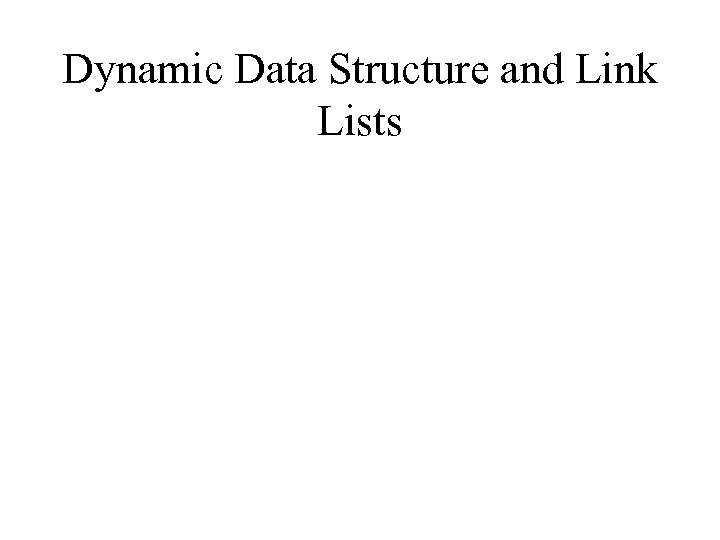Dynamic Data Structure and Link Lists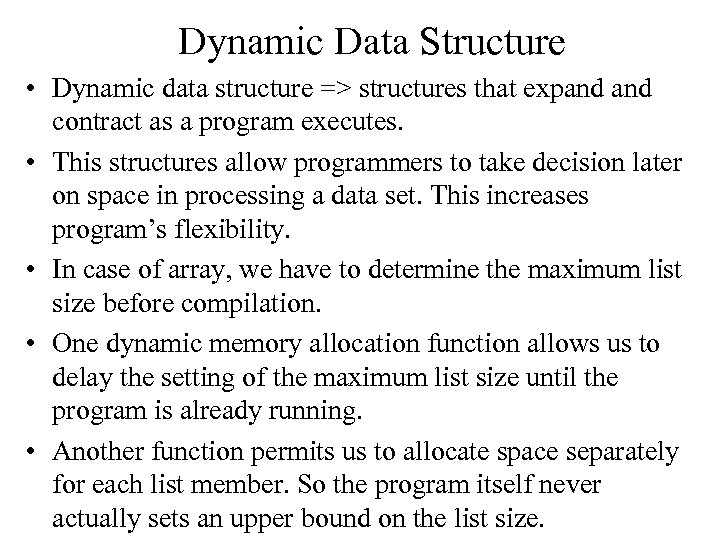Dynamic Data Structure • Dynamic data structure => structures that expand contract as a program executes. • This structures allow programmers to take decision later on space in processing a data set. This increases program’s flexibility. • In case of array, we have to determine the maximum list size before compilation. • One dynamic memory allocation function allows us to delay the setting of the maximum list size until the program is already running. • Another function permits us to allocate space separately for each list member. So the program itself never actually sets an upper bound on the list size.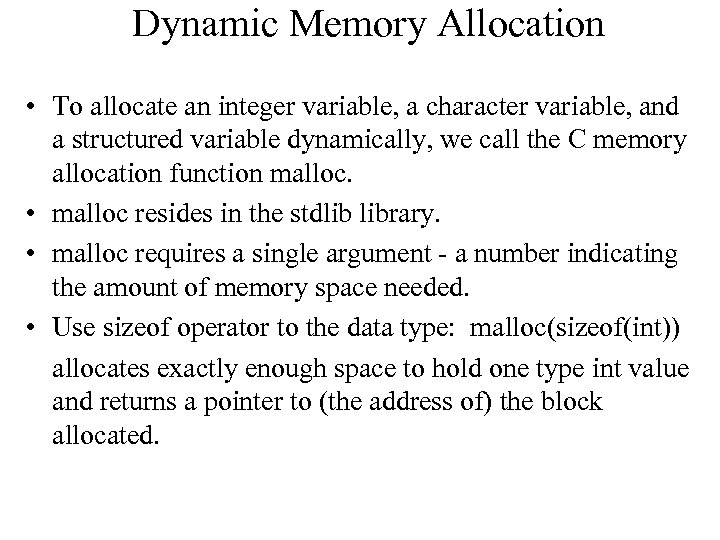Dynamic Memory Allocation • To allocate an integer variable, a character variable, and a structured variable dynamically, we call the C memory allocation function malloc. • malloc resides in the stdlib library. • malloc requires a single argument - a number indicating the amount of memory space needed. • Use sizeof operator to the data type: malloc(sizeof(int)) allocates exactly enough space to hold one type int value and returns a pointer to (the address of) the block allocated.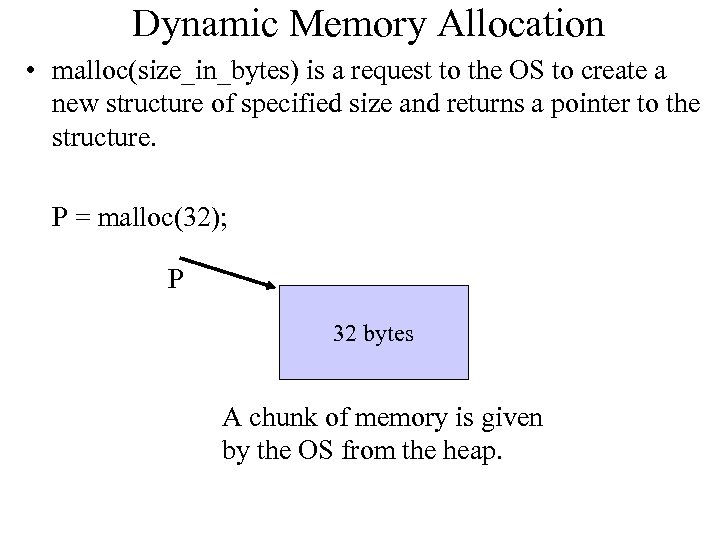Dynamic Memory Allocation • malloc(size_in_bytes) is a request to the OS to create a new structure of specified size and returns a pointer to the structure. P = malloc(32); P 32 bytes A chunk of memory is given by the OS from the heap.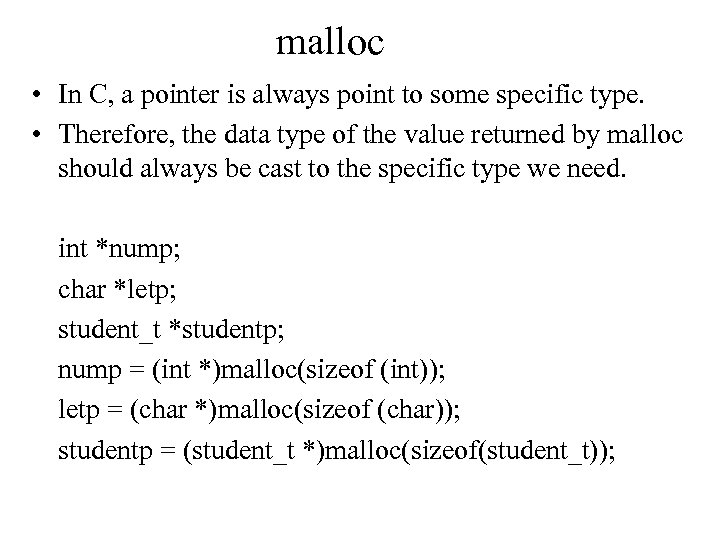malloc • In C, a pointer is always point to some specific type. • Therefore, the data type of the value returned by malloc should always be cast to the specific type we need. int *nump; char *letp; student_t *studentp; nump = (int *)malloc(sizeof (int)); letp = (char *)malloc(sizeof (char)); studentp = (student_t *)malloc(sizeof(student_t));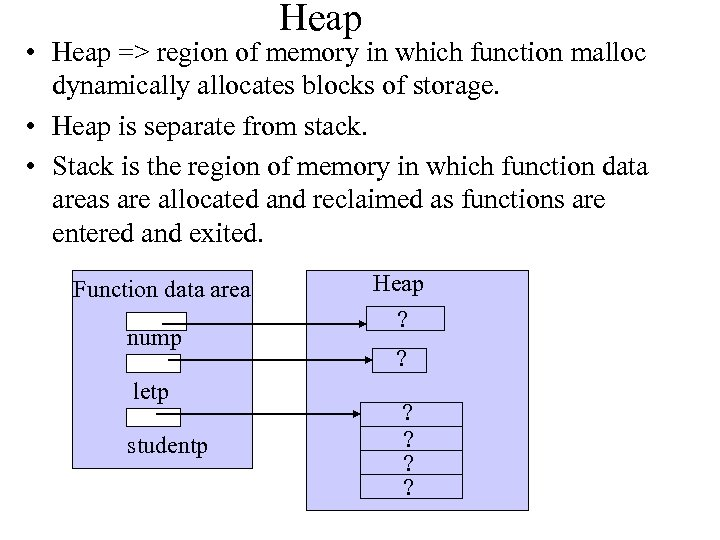Heap • Heap => region of memory in which function malloc dynamically allocates blocks of storage. • Heap is separate from stack. • Stack is the region of memory in which function data areas are allocated and reclaimed as functions are entered and exited. Function data area nump letp studentp Heap ? ? ?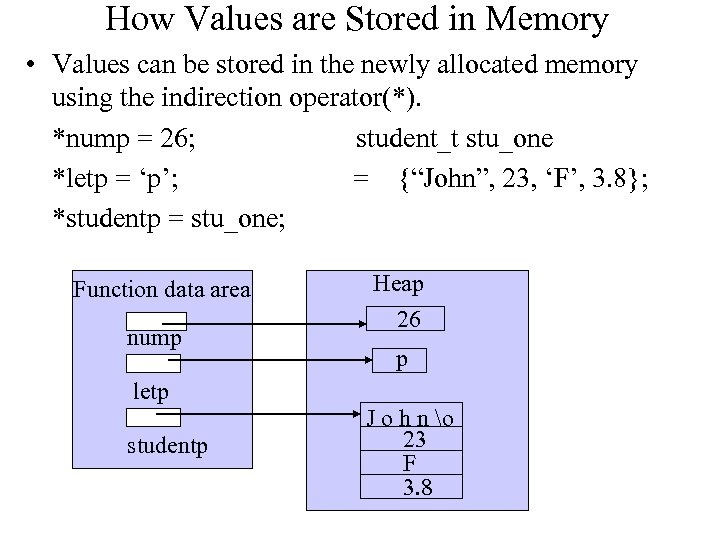How Values are Stored in Memory • Values can be stored in the newly allocated memory using the indirection operator(*). *nump = 26; student_t stu_one *letp = ‘p’; = {“John”, 23, ‘F’, 3. 8}; *studentp = stu_one; Function data area nump Heap 26 p letp studentp J o h n o 23 F 3. 8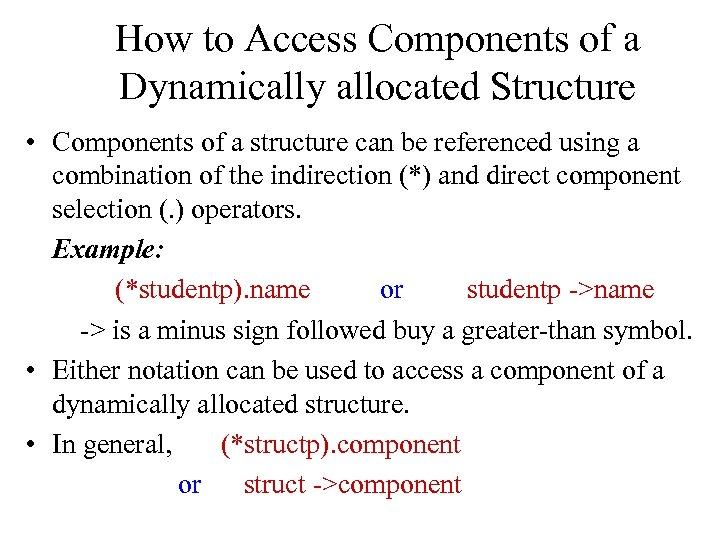How to Access Components of a Dynamically allocated Structure • Components of a structure can be referenced using a combination of the indirection (*) and direct component selection (. ) operators. Example: (*studentp). name or studentp ->name -> is a minus sign followed buy a greater-than symbol. • Either notation can be used to access a component of a dynamically allocated structure. • In general, (*structp). component or struct ->component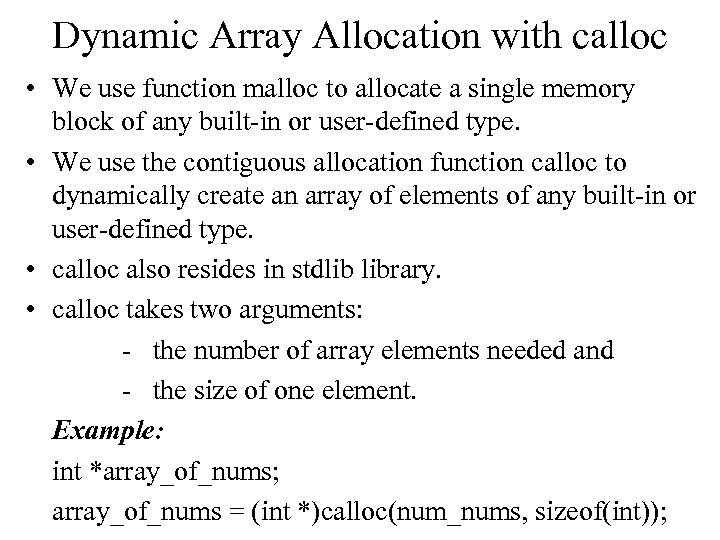Dynamic Array Allocation with calloc • We use function malloc to allocate a single memory block of any built-in or user-defined type. • We use the contiguous allocation function calloc to dynamically create an array of elements of any built-in or user-defined type. • calloc also resides in stdlib library. • calloc takes two arguments: - the number of array elements needed and - the size of one element. Example: int *array_of_nums; array_of_nums = (int *)calloc(num_nums, sizeof(int));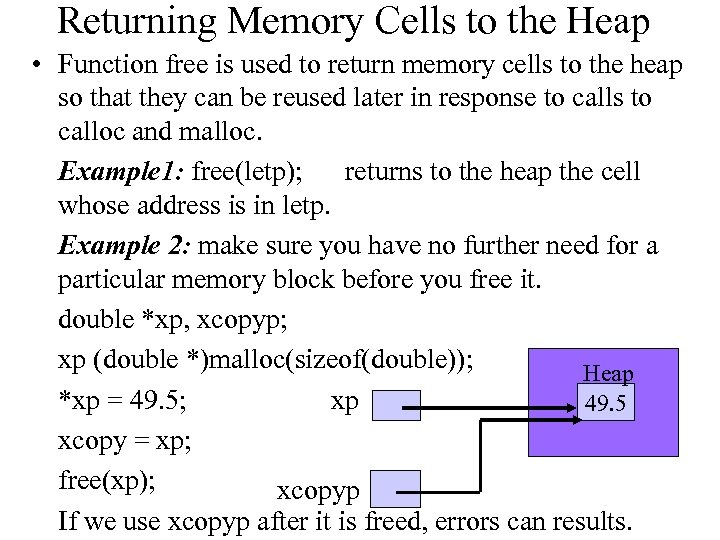Returning Memory Cells to the Heap • Function free is used to return memory cells to the heap so that they can be reused later in response to calls to calloc and malloc. Example 1: free(letp); returns to the heap the cell whose address is in letp. Example 2: make sure you have no further need for a particular memory block before you free it. double *xp, xcopyp; xp (double *)malloc(sizeof(double)); Heap xp *xp = 49. 5; 49. 5 xcopy = xp; free(xp); xcopyp If we use xcopyp after it is freed, errors can results.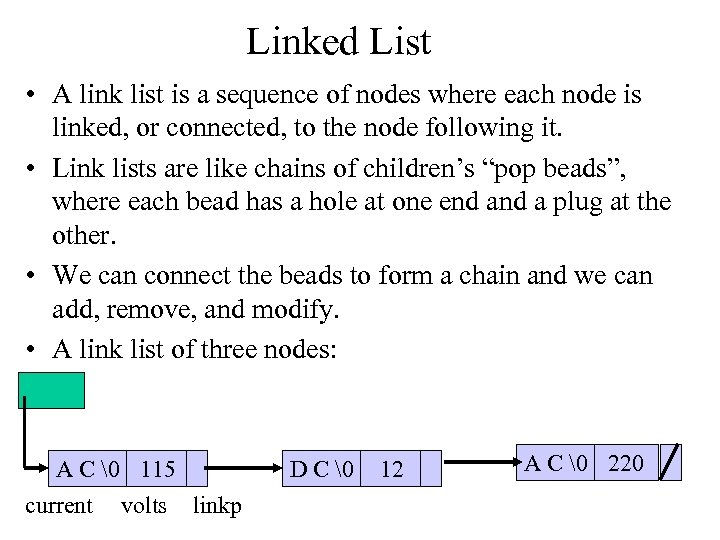Linked List • A link list is a sequence of nodes where each node is linked, or connected, to the node following it. • Link lists are like chains of children’s “pop beads”, where each bead has a hole at one end a plug at the other. • We can connect the beads to form a chain and we can add, remove, and modify. • A link list of three nodes: A C 115 current volts linkp D C 12 A C 220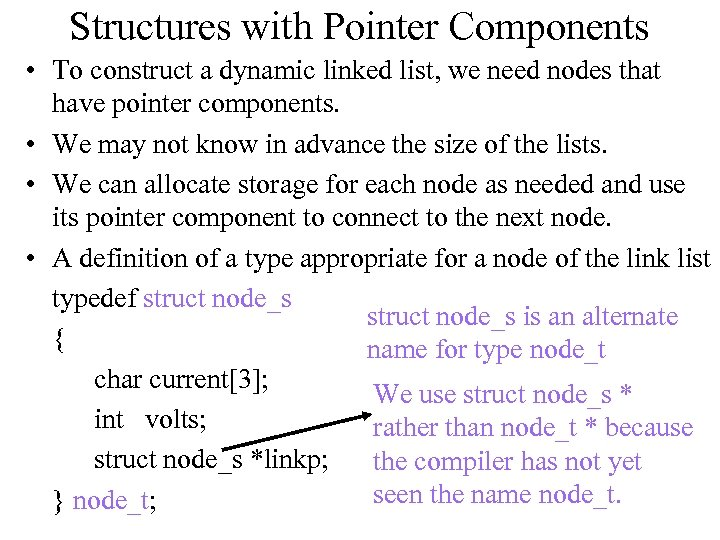Structures with Pointer Components • To construct a dynamic linked list, we need nodes that have pointer components. • We may not know in advance the size of the lists. • We can allocate storage for each node as needed and use its pointer component to connect to the next node. • A definition of a type appropriate for a node of the link list typedef struct node_s is an alternate { name for type node_t char current; We use struct node_s * int volts; rather than node_t * because struct node_s *linkp; the compiler has not yet seen the name node_t. } node_t;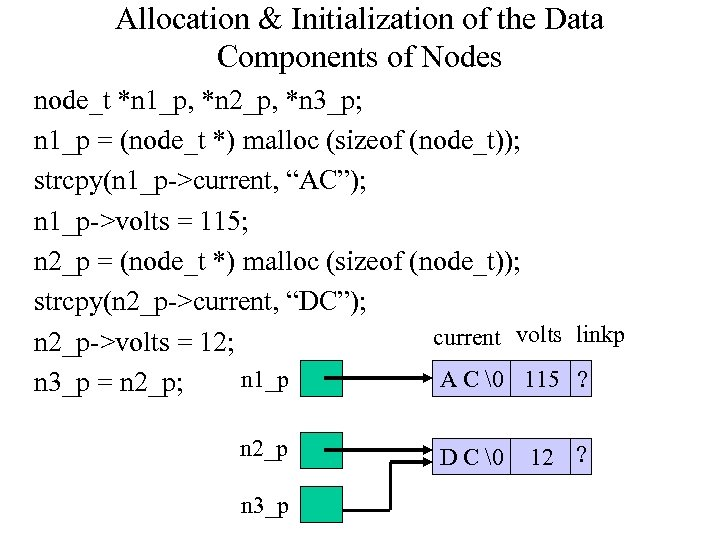Allocation & Initialization of the Data Components of Nodes node_t *n 1_p, *n 2_p, *n 3_p; n 1_p = (node_t *) malloc (sizeof (node_t)); strcpy(n 1_p->current, “AC”); n 1_p->volts = 115; n 2_p = (node_t *) malloc (sizeof (node_t)); strcpy(n 2_p->current, “DC”); current volts linkp n 2_p->volts = 12; n 1_p A C 115 ? n 3_p = n 2_p; n 2_p n 3_p D C 12 ?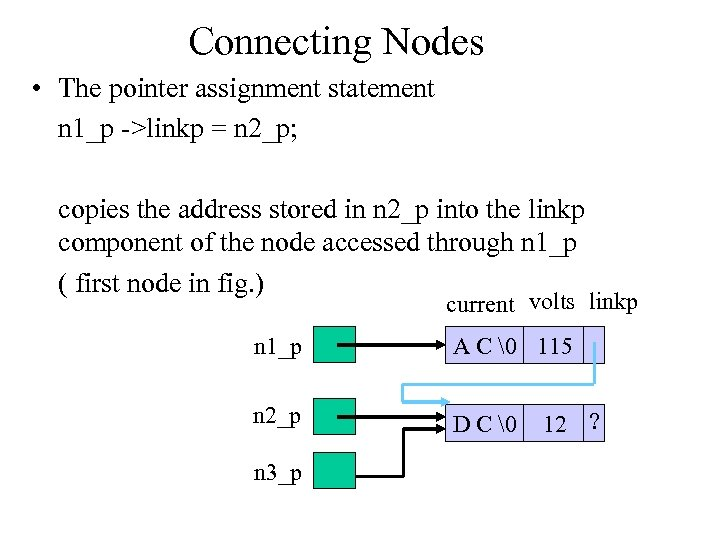Connecting Nodes • The pointer assignment statement n 1_p ->linkp = n 2_p; copies the address stored in n 2_p into the linkp component of the node accessed through n 1_p ( first node in fig. ) current volts linkp n 1_p A C 115 n 2_p D C n 3_p 12 ?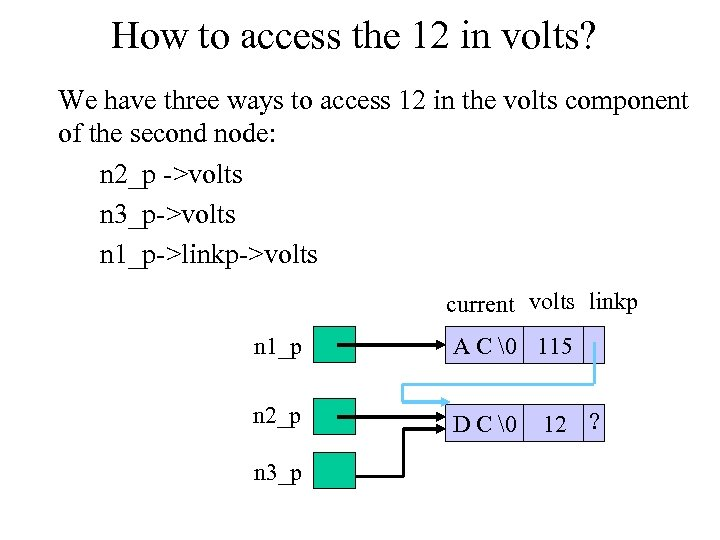How to access the 12 in volts? We have three ways to access 12 in the volts component of the second node: n 2_p ->volts n 3_p->volts n 1_p->linkp->volts current volts linkp n 1_p A C 115 n 2_p D C n 3_p 12 ?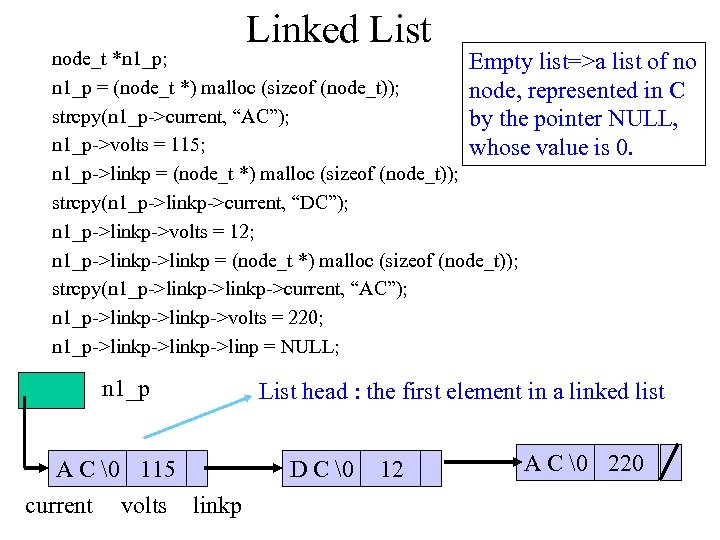Linked List node_t *n 1_p; Empty list=>a list of no n 1_p = (node_t *) malloc (sizeof (node_t)); node, represented in C strcpy(n 1_p->current, “AC”); by the pointer NULL, n 1_p->volts = 115; whose value is 0. n 1_p->linkp = (node_t *) malloc (sizeof (node_t)); strcpy(n 1_p->linkp->current, “DC”); n 1_p->linkp->volts = 12; n 1_p->linkp = (node_t *) malloc (sizeof (node_t)); strcpy(n 1_p->linkp->current, “AC”); n 1_p->linkp->volts = 220; n 1_p->linkp->linp = NULL; n 1_p A C 115 current volts linkp List head : the first element in a linked list D C 12 A C 220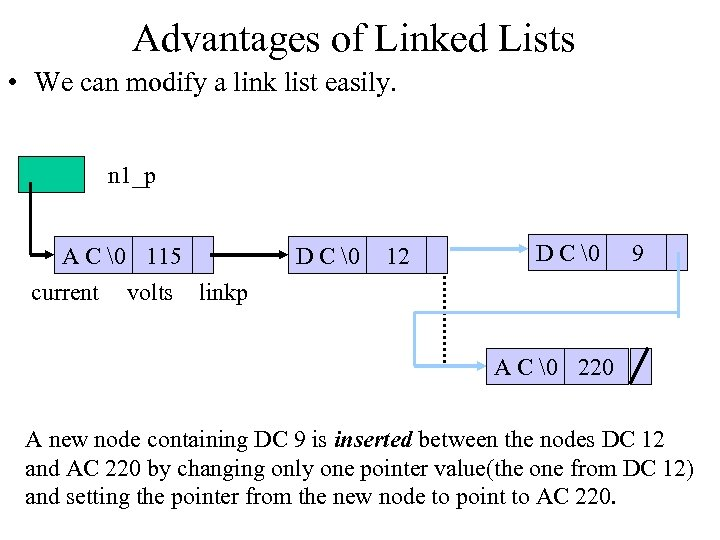Advantages of Linked Lists • We can modify a link list easily. n 1_p A C 115 current volts linkp D C 12 D C 9 A C 220 A new node containing DC 9 is inserted between the nodes DC 12 and AC 220 by changing only one pointer value(the one from DC 12) and setting the pointer from the new node to point to AC 220.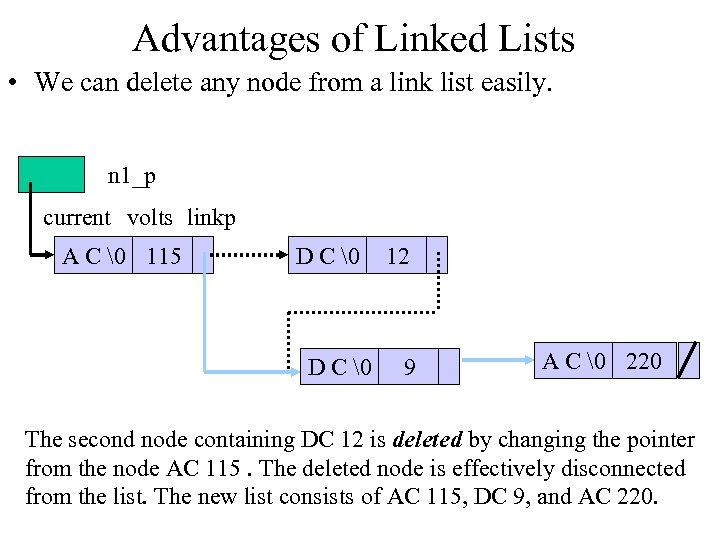Advantages of Linked Lists • We can delete any node from a link list easily. n 1_p current volts linkp A C 115 D C 12 9 A C 220 The second node containing DC 12 is deleted by changing the pointer from the node AC 115. The deleted node is effectively disconnected from the list. The new list consists of AC 115, DC 9, and AC 220.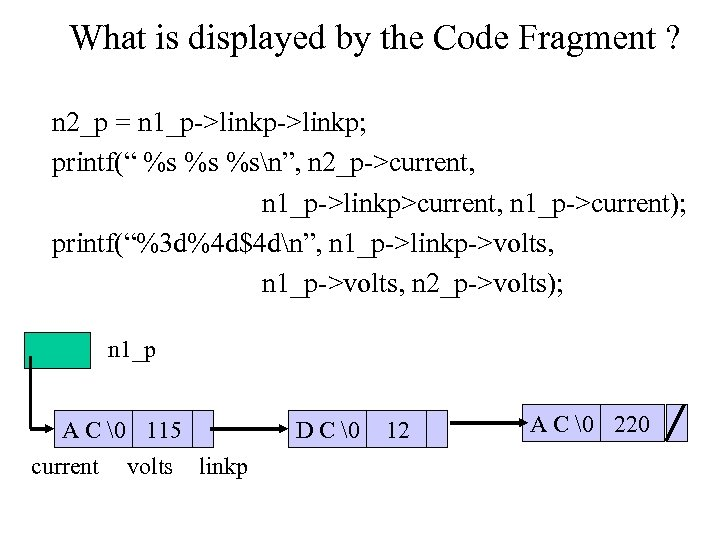What is displayed by the Code Fragment ? n 2_p = n 1_p->linkp; printf(“ %s %s %sn”, n 2_p->current, n 1_p->linkp>current, n 1_p->current); printf(“%3 d%4 d\$4 dn”, n 1_p->linkp->volts, n 1_p->volts, n 2_p->volts); n 1_p A C 115 current volts linkp D C 12 A C 220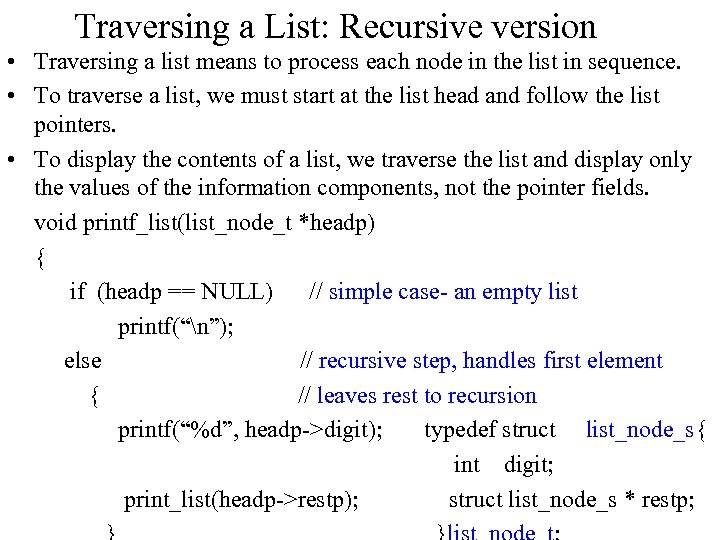Traversing a List: Recursive version • Traversing a list means to process each node in the list in sequence. • To traverse a list, we must start at the list head and follow the list pointers. • To display the contents of a list, we traverse the list and display only the values of the information components, not the pointer fields. void printf_list(list_node_t *headp) { if (headp == NULL) // simple case- an empty list printf(“n”); else // recursive step, handles first element { // leaves rest to recursion printf(“%d”, headp->digit); typedef struct list_node_s{ int digit; print_list(headp->restp); struct list_node_s * restp;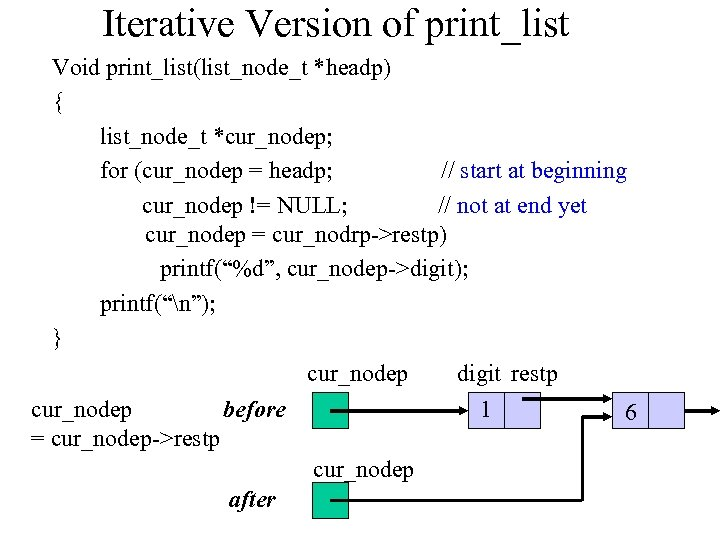Iterative Version of print_list Void print_list(list_node_t *headp) { list_node_t *cur_nodep; for (cur_nodep = headp; // start at beginning cur_nodep != NULL; // not at end yet cur_nodep = cur_nodrp->restp) printf(“%d”, cur_nodep->digit); printf(“n”); } cur_nodep digit restp before cur_nodep = cur_nodep->restp 1 cur_nodep after 6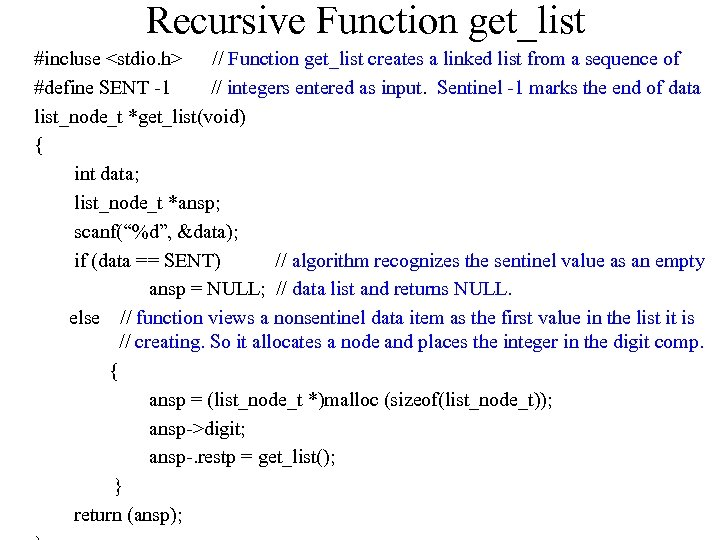Recursive Function get_list #incluse // Function get_list creates a linked list from a sequence of #define SENT -1 // integers entered as input. Sentinel -1 marks the end of data list_node_t *get_list(void) { int data; list_node_t *ansp; scanf(“%d”, &data); if (data == SENT) // algorithm recognizes the sentinel value as an empty ansp = NULL; // data list and returns NULL. else // function views a nonsentinel data item as the first value in the list it is // creating. So it allocates a node and places the integer in the digit comp. { ansp = (list_node_t *)malloc (sizeof(list_node_t)); ansp->digit; ansp-. restp = get_list(); } return (ansp);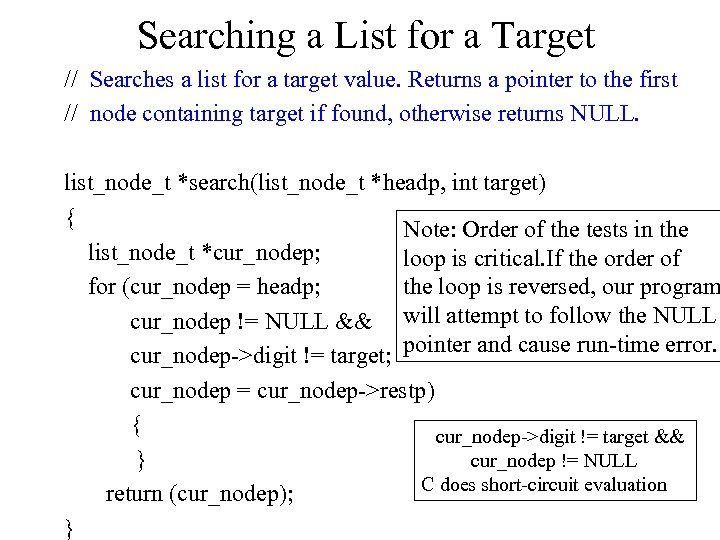Searching a List for a Target // Searches a list for a target value. Returns a pointer to the first // node containing target if found, otherwise returns NULL. list_node_t *search(list_node_t *headp, int target) { Note: Order of the tests in the list_node_t *cur_nodep; loop is critical. If the order of for (cur_nodep = headp; the loop is reversed, our program cur_nodep != NULL && will attempt to follow the NULL cur_nodep->digit != target; pointer and cause run-time error. cur_nodep = cur_nodep->restp) { cur_nodep->digit != target && cur_nodep != NULL } C does short-circuit evaluation return (cur_nodep); }